Instructions

For the following questions answer them individually

Question 1

# Consider an expanding sphere of instantaneous radius R whose total mass remains constant. The expansion is such that the instantaneous density $$\rho$$ remains uniform throughout the volume. The rate of fractional change in density $$(\frac{1}{\rho}\frac{d\rho}{dt})$$ is constant. The velocity $$\nu$$ of any point on the surface of the expanding sphere is proportional toQuestion 2

# Consider regular polygons with number of sides n=3,4,5.....as shown in the figure. The center of mass of all the polygons is at height h from the ground. They roll on a horizontal surface about the leading vertex without slipping and sliding as depicted. The maximum increase in height of the locus of the center of mass for each polygon is $$\triangle$$. Then $$\triangle$$ depends on n and has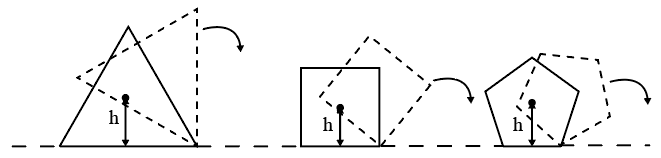Question 3

# A photoelectric material having work-function $$\phi_{0}$$ is illuminated with light of wavelength $$\lambda (\lambda < \frac{hc}{\phi_{0}})$$. The fastest photoelectron has a de Broglie wavelength $$\lambda_{d}$$. A change in wavelength of the incident light by $$\triangle \lambda$$ results in a change $$\triangle \lambda_{d}$$ in $$\lambda_{d}$$. Then the ratio $$\frac{\triangle\lambda_{d}}{\triangle\lambda}$$ is proportional toQuestion 4

# A symmetric star shaped conducting wire loop is carrying a steady state current I as shown in the figure. The distance between the diametrically opposite vertices of the star is $$4a$$. The magnitude of the magnetic field at the center of the loop is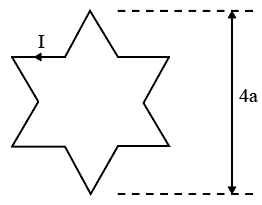Question 5

# Three vectors $$\overrightarrow{P},\overrightarrow{Q}$$ and $$\overrightarrow{R}$$ are shown in the figure. Let be any point on the vector $$\overrightarrow{R}$$. The distance between the points $$P$$ and $$S$$ is $$b\mid\overrightarrow{R}\mid$$. The general relation among vectors $$\overrightarrow{P},\overrightarrow{Q}$$ and $$\overrightarrow{S}$$ is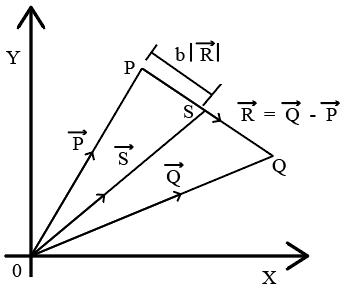Question 6

# A rocket is launched normal to the surface of the Earth, away from the Sun, along the line joining the Sun and the Earth. The Sun is $$3\times10^{5}$$ times heavier than the Earth and is at a distance $$2.5\times10^{4}$$ times larger than the radius of the Earth. The escape velocity from Earth’s gravitational field is$$\nu_{e}=11.2 kms^{-1}$$. The minimum initial velocity $$\nu_{s}$$ required for the rocket to be able to leave the Sun-Earth system is closest to(Ignore the rotation and revolution of the Earth and the presence of any other planet)Question 7

# A person measures the depth of a well by measuring the time interval between dropping a stone and receiving the sound of impact with the bottom of the well. The error in his measurement of time is $$\delta T=0.01$$ seconds and he measures the depth of the well to be $$L=20$$ meters. Take the acceleration due to gravity $$g = 10 ms^{-2}$$ and the velocity of sound is $$300 ms^{-1}$$. Then the fractional error in the measurement, $$\frac{\delta L}{L}$$, is closest toQuestion 8

# A uniform magnetic field B exists in the region between $$x=0$$ and $$x=\frac{3R}{2}$$ (region 2 in the figure) pointing normally into the plane of the paper. A particle with charge $$+Q$$ and momentum $$p$$ directed along x-axis enters region 2 from region 1 at point $$P_{1}(Y=-R)$$.Which of the following option(s) is/are correct?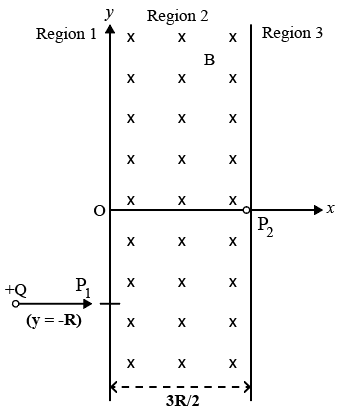Question 9

# The instantaneous voltages at three terminals marked X,Y and Z are given by $$V_{X}= V_{0} \sin \omega t$$, $$V_{Y}= V_{0} \sin (\omega t+\frac{2\pi}{3})$$and $$V_{Z}= V_{0} \sin (\omega t+\frac{4\pi}{3})$$. An ideal voltmeter is configured to read rms value of the potential difference between its terminals. It is connected between points X and Y and then between Y and Z The reading(s) of the voltmeter will beQuestion 10

# A point charge $$+Q$$ is placed just outside an imaginary hemispherical surface of radius R as shown in the figure. Which of the following statements is/are correct?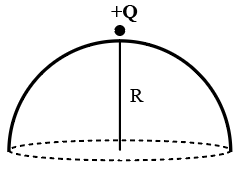OR